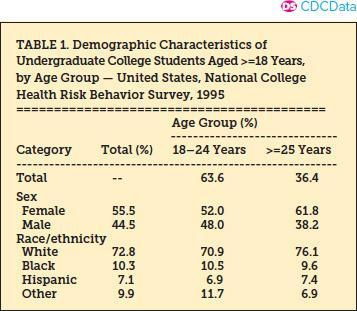voyage

Please answer each of the following questions in detail and provide examples for better clarity wherever applicable. Include in-text citations.

1. Define each of the following terms and provide an example for each:

1. An event, union of events, intersection of events, sample space, mutually exclusive events, the complement of an event, containment, the null event, disjoint events, the probability of an event, probability space, probability by counting, probability of union of events, probability of union of disjoint events, the relation between probabilities of complementary events, relation between the probabilities of the contained event and the probability of its container.
2. Please explain conditional probability accompanied by an example based on a contingency table. Explain how the notion of conditional probability yields the relation for the probability of intersection of independent events.
3. Please explain the Bayes’ theorem accompanied by an example.
4. Demographic characteristics of college students in the USA whose ages are at least 18, according to a survey taken in 1995 are shown in Table 1. Using conditional probabilities, discuss:
5. The dependence between age and gender
6. The dependence between age and ethnicity for U.S. college students

Table 1

Demographic characters of college students in the USA whose ages are at least 18 in 1995.Note:

Note:

1. Need to have at least 1 peer-reviewed article as the reference and textbook as the reference

2. Need in-text citation

3. Please find the attachments as the power points of the course for reference.

4. Textbook Information:

Bowerman, B., Drougas, A. M., Duckworth, A. G., Hummel, R. M. Moniger, K. B., & Schur, P. J.  (2019). Business statistics and analytics in practice (9th ed.). McGraw-Hill

ISBN 9781260187496

5. Please find the Course Learning Outcome list of this course in the attachment

• 10 days ago
• 5
••••